How to subtotal in excel, with two fields, copy, sort, remove the results of subtotal

Lionsure 2019-11-23 Original by the website

You can subtotal with the function of subtotal, PivotTable, and formula in Excel. You can subtotal in the original tabl with the function of subtotal. It can complete the subtotal of one field, two fields or more than two fields, but sort before subtotaling, and it is not flexible and cannot complete complicated subtotal. You must create a PivotTable before subtotaling, if you subtotal with PivotTable. It is powerful, simple to subtotal, and can be aggregated to meet various requirements. The formula is flexible and can subtotal according to various criteria, and the results of subtotal can be extracted, but the formula is complicated. This article will first introduce the subtotal by the function of subtotal, and the other two subtotal methods are introduced in the next article.

The operations with function of subtotal in Excel are divided into the subtotal for the fields to be selected, the results of subtotal are copied, sorted and deleted. Sometimes you may encounter that sort and subtotal are grayed out when subtotal, what is the reason? Let's start with the discussion of subtotals from this issue.

I. Why are the sort, filter and subtotal grayed out in excel?(Excel subtotal not available)

1. Click the column number H, select the column H, select the "Data" tab, the Sort, Filter and subtotal are grayed out; select the range in which there are data, the Sort and Filter are available, but the subtotal is still not available; the demo is shown in Figure 1:Figure 1

2. The solution. Right-click one of the cells in the table, select "Table → Convert To Range" in the pop-up menu, pop-up a dialog box that asks "Do you want to convert the table to normal range", click "Yes", then the table will be converted to range, sort, filter And subtotal are available; the process steps are shown in Figure 2: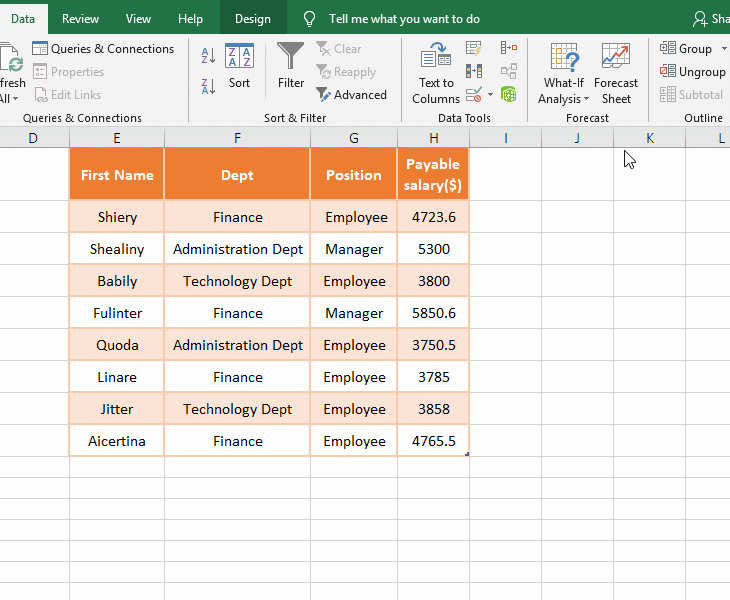Figure 2

II, How to subtotal in excel

(I) How to insert subtotals in excel by one field

If you want to subtotal by department, calculate the "Payable Salary" for each department. The operation method is as follows:

1. Sort. Click the column number B, select the column B, select the "Data" tab, click the "A → Z" icon, open the "Sort Warning" dialog box, select "Extend the selection", click "Sort", then column B is sorted in ascending order, and the same departments are connected together, so that it is convenient to subtotal the same department; the operation process steps are as shown in Figure 3: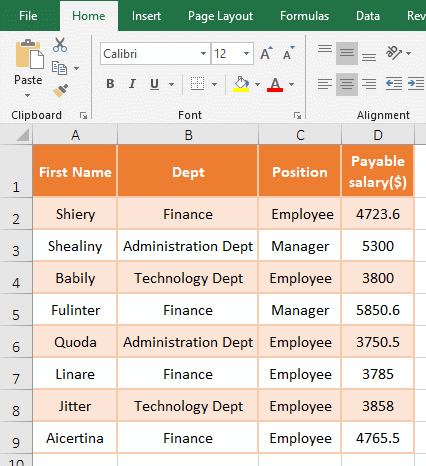Figure 3

2. Subtotal. Select any cell in the table, hold down Alt, press A and B once(or select the "Data" tab, click "Subtotal" in the top right of the screen), open the "Subtotal" dialog, select "Dept" for "At each change in", select "Sum" for "Use function", check the "Payable Salary" for "Add subtotal to", and other keep the default settings, click "OK" to subtotal the ""Payable Salary"" of each department; Operation process steps, see screenshot in Figure 4: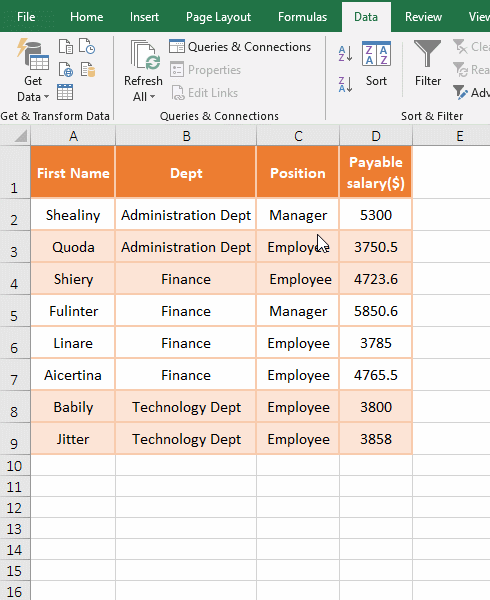Figure 4

The "Replace Current Subtotals" is used to replace the old subtotals with the current subtotals.

The "Summary below data" means that the results of subtotal are displayed below each type of data. For example, the results of subtotal are displayed below each department in the demo; if it is not selected, the results of subtotal are displayed above each type of data.

The "Page break between groups" means to display each group of data on one page; for example, subtotal by "department", the data of each department is displayed on one page. The demonstration is shown in Figure 5: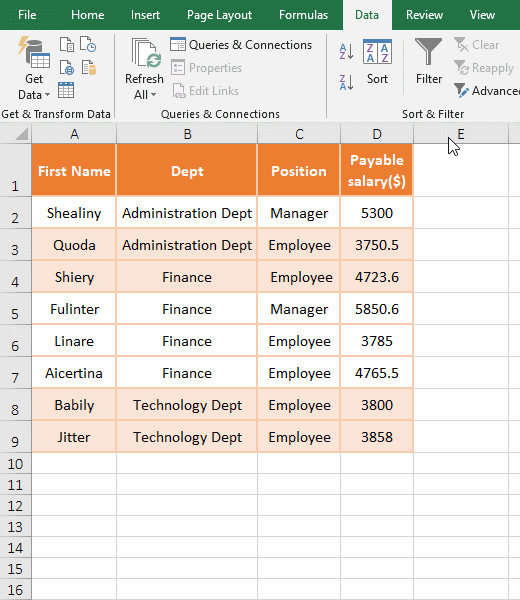Figure 5

Tip: When you subtotal, If it prompts "Microsoft Excel cannot determine which row in your list or selection contains column labels, which are required for this command.", because the header row in table has merged cells, split the merged cells to solve.

(II) How to do multiple subtotals in excel(How to subtotal groups in excel)

If you want to subtotal by the two fields of "Clothes and City", calculate the sales volume of each clothing and each city. The operation method is as follows:

1. Sort the two fields "Clothes and city" to be subtotaled as "Primary Key and Secondary Key". Select one of the cells in the table, select the "Data" tab, click "Sort", open the "Sort" dialog box, select "Clothes" for "Sort by", click "Add Level" to add a condition, select "City" for "Then by", click "OK", then sort by "Clothes and City" in ascending order; the operation process steps, see screenshot in Figure 6: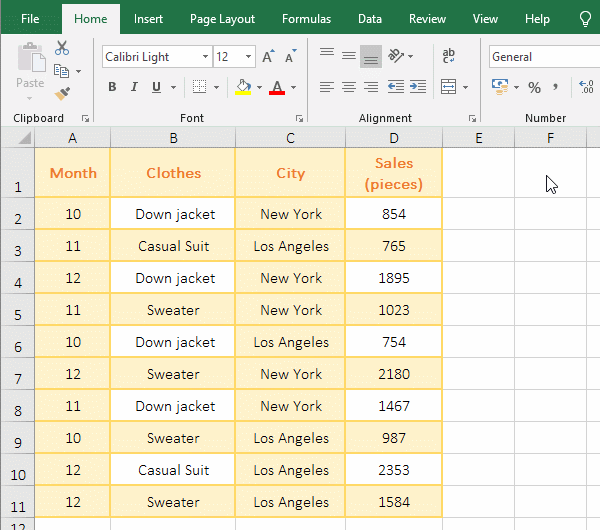Figure 6

2. Subtotals. Select one of the cells in the table, hold down Alt, press A and B once, open the "Subtotal" dialog box, select "Clothes" for "At each change in", other keep the default settings, and click "OK" to subtotal "Sales" of each type of clothing; press Alt again, press A and B respectively, open the "Subtotal" dialog box again, select "City for "At each change in", uncheck "Replace current subtotals", click "OK" ", then subtotal the sales volume of each city; the operation process steps, see screenshot in Figure 7: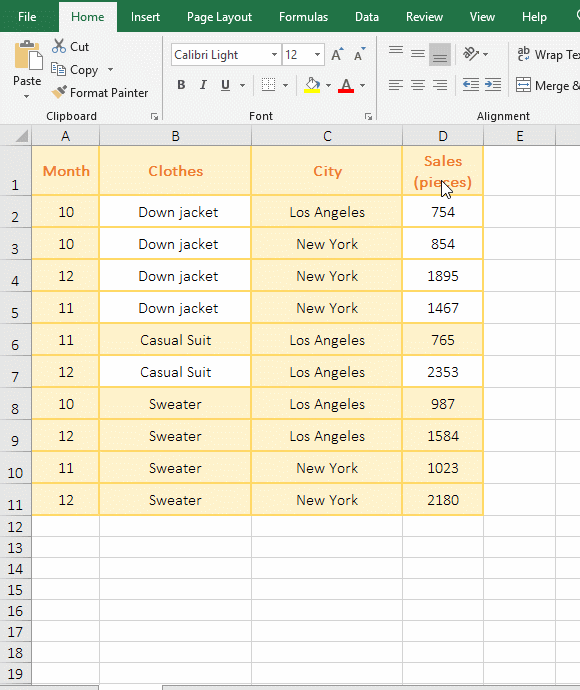Figure 7

3. Group browsing. Click 3 in the top left of the screen to display the results of subtotal by "Clothes and City", and then click 2 to display only the results of subtotal by "Clothes"; the operation steps are as shown in Figure 8: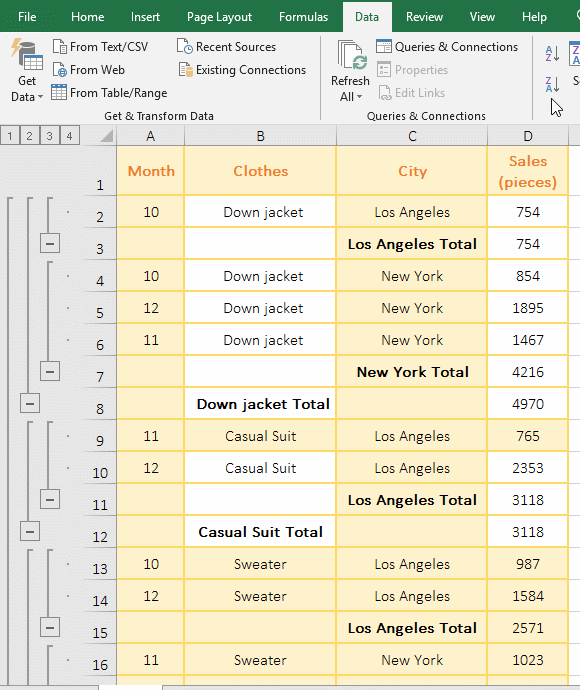Figure 8

III, How to copy and paste subtotals in excel

1. Click 3 in the top left of the screen to display only the results of subtotal. Click A1 to select it, hold Shift, click the last cell D20 in the bottom right of the data range, and select the data range. Press F5 to open the "Go To" dialog box, click "Special", in the dialog box that has been opened, select "Visible cell only", click "OK", select all visible cells in the data range, press Ctrl + C to copy;

2. Click the worksheet label "Subtotal results" to switch to the worksheet, press Ctrl + V, then only the results of subtotal will be copied to the new table; the operation steps are as shown in Figure 9: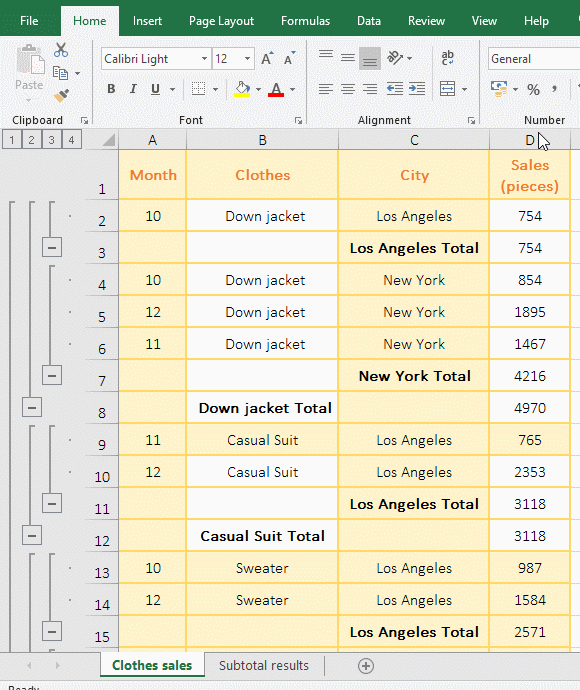Figure 9

IV, How to sort subtotals in excel

1. Click 2 in the upper left corner of the screen to display only the results subtotaled by department, click the column number D to select the column D, select the "Data" tab, and click the "A → Z" icon to open the "Sort Warning" dialog box, select "Extended the selection", click "Sort", then the results of subtotal are sorted in ascending order according to "Payable salary(\$)". Click 3 in the top left of the screen to display all the contents, and each row of data is also sorted with the results of subtotal. The operation process steps are shown in Figure 10: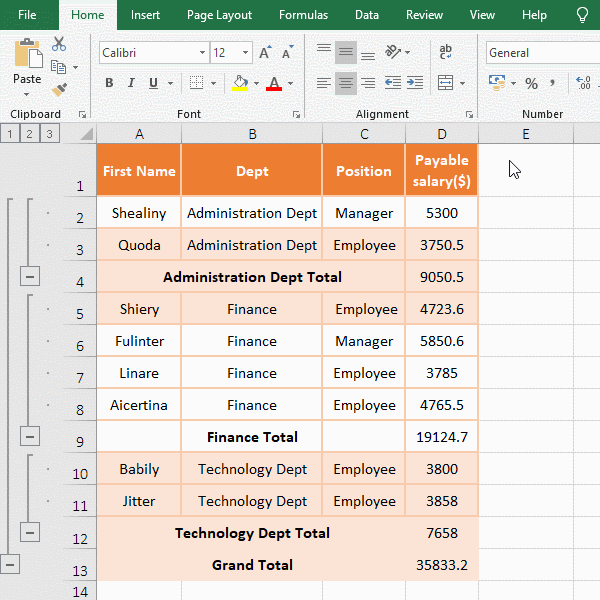Figure 10

2. If you subtotal by the two fields, only the results of the first field(ie, the level 2 of the group) can be sorted.

V, How to remove subtotals in excel

1. Select any cell in the table, press and hold Alt, press A and B respectively to open the "Subtotals" dialog box, and click "Remove All", the results of subtotal are deleted; the operation steps are as shown in Figure 11: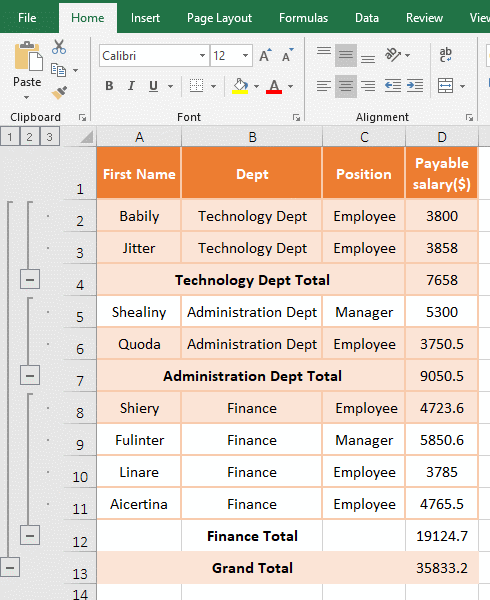Figure 11

2. Tip: If you can't delete it with "Remove All", close and re-open Excel and delete it again; if you still can't delete it, close all open Excel and then re-open it; if not, restart the operating system and try to delete it; If it still doesn't work, use the "group browsing" method above to display only the results of subtotal, then hold down Alt, select the results of subtotal in a row, be careful not to select the hidden rows, all right, right click them, select "Delete" in the pop-up menu.

If you can't complete the required subtotal with the function of subtotal in Excel, you can use the PivotTable and the formula. For details, refer to the article "Eexcel subtotal by year, month and week with Pivot Table and Formula, and extract results of subtota".